# Wackano Extended

## 1 Introduction

• Using a Mechanisms set, build the following mechanisms.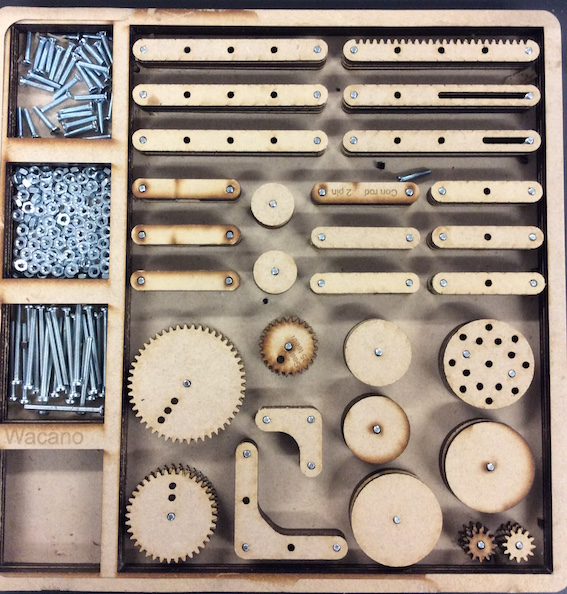• Below is a picture of a Bell crank linkage, build it using the Mechanisms set.
• Discuss how the Bell linkage changes the direction of the initial movement.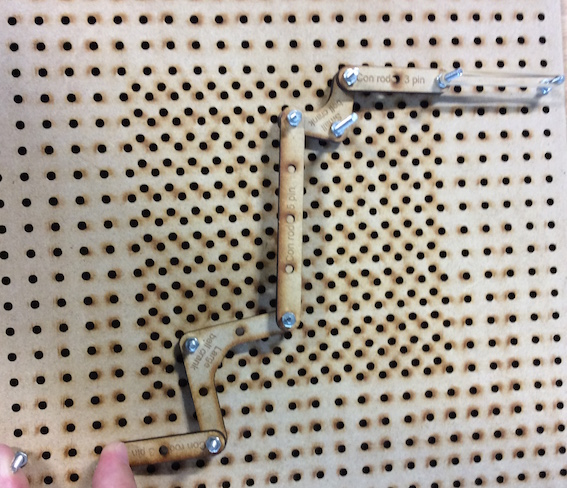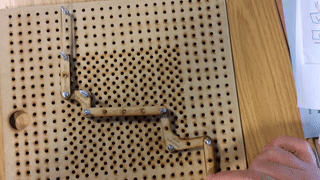• Below is a picture of a Treadle linkage, build it using the Mechanisms set.
• Discuss how the Treadle linkage changes the direction of the initial movement.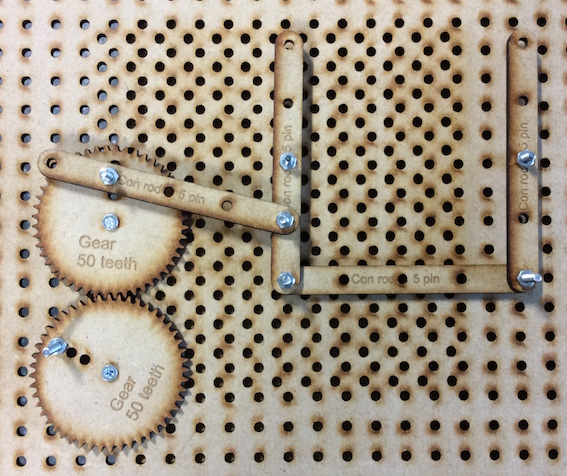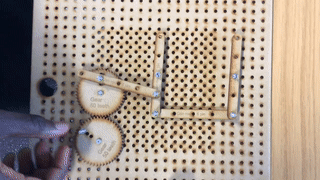### Gearing

• Below is a picture of a Gear System, build it using the Mechanisms set.
• Discuss how the gear system changes the direction of each gear in the system.
• Calculate the ratios of each gear in the system.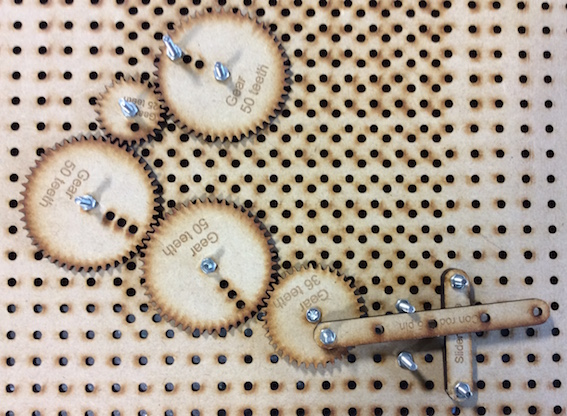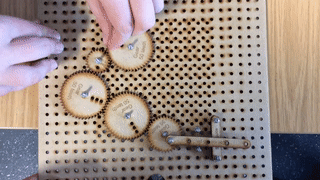• Be creative and design your own gear system.
• Now calculate out the gear ratios of each gear.

### Challenge

• Using the skills you have learned from building the Wackano mechanisms. Design a system which has an object moving in each of the far corners of the board. All the objects must move from one point.